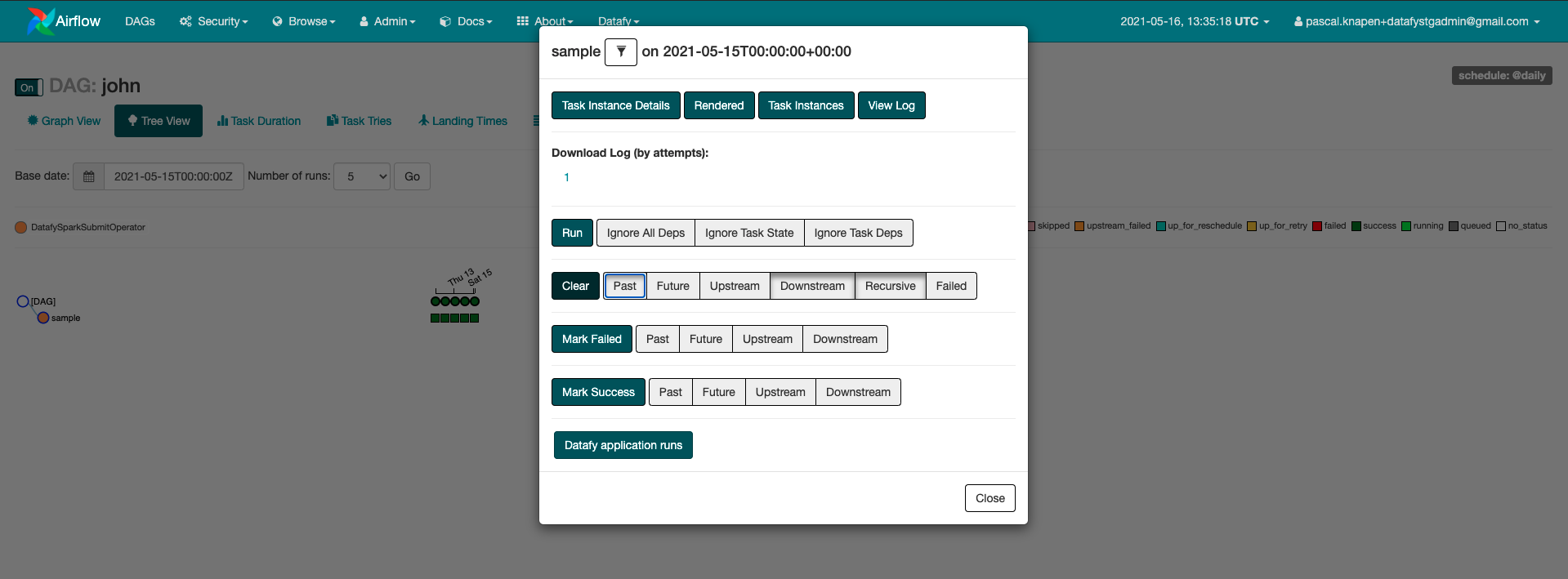# 6. Run against real data

Everything is now in place to update the code and run the project against real data. Open the project in your favourite Python IDE. For example, PyCharm or VS Code.

## 6.1. Update the code​

In this section, we will update the code to:

1. Export the data from the public OpenAQ dataset
2. Transform the data by adding a datestamp column and filter on Belgium data
3. Load the data into S3 and register it in the Glue catalog

Open the `app.py` file and replace it with the content below.

caution

Don't forget to replace PROJECT_NAME with your project name and GLUE_DATABASE with the name of the Glue database created in the previous step.

If you specified a GLUE_DATABASE with another name than `default`, you should make sure the `default` database also exists otherwise Spark will try to create it, but will not have the necessary permissions based on the created IAM role.

``import argparsefrom pyspark.sql import SparkSessionfrom pyspark.sql.dataframe import DataFramefrom PROJECT_NAME.common.spark import ClosableSparkSession, SparkLoggerfrom PROJECT_NAME.transformations.shared import add_ds, filter_by_countrydef main():    parser = argparse.ArgumentParser(description="PROJECT_NAME")    parser.add_argument(        "-d", "--date", dest="date", help="date in format YYYY-mm-dd", required=True    )    parser.add_argument(        "-e", "--env", dest="env", help="environment we are executing in", required=True    )    args = parser.parse_args()    with ClosableSparkSession("PROJECT_NAME") as session:        run(session, args.env, args.date)def run(spark: SparkSession, environment: str, date: str):    """Main ETL script definition.    :return: None    """    # execute ETL pipeline    logger = SparkLogger(spark)    logger.info(f"Executing job for {environment} on {date}")    data = extract_data(spark, date)    transformed = transform_data(data, date)    load_data(spark, transformed)def extract_data(spark: SparkSession, date: str) -> DataFrame:    """Load data from a source    :param spark: Spark session object.    :param date: The execution date as a string    :return: Spark DataFrame.    """    return spark.read.json(f"s3://openaq-fetches/realtime-gzipped/{date}")def transform_data(data: DataFrame, date: str) -> DataFrame:    """Transform the original dataset.    :param data: Input DataFrame.    :param date: The context date    :return: Transformed DataFrame.    """    return data.transform(add_ds(date)).transform(filter_by_country("BE"))def load_data(spark: SparkSession, data: DataFrame) -> None:    """Writes the output dataset to some destination    :param spark: The spark session    :param data: DataFrame to write.    :return: None    """    spark.catalog.setCurrentDatabase(f"GLUE_DATABASE")    (data.coalesce(1)        .write        .partitionBy("ds")        .mode("overwrite")        .format("parquet")        .saveAsTable("PROJECT_NAME")    )if __name__ == "__main__":    main()``

Replace the file `transformations/shared.py`

``from pyspark.sql.dataframe import DataFramefrom pyspark.sql.functions import litfrom datetime import datetimedef add_ds(date: str):    actual_date = datetime.strptime(date, "%Y-%m-%d").date()    def inner(df: DataFrame):        return df.withColumn("ds", lit(actual_date))    return innerdef filter_by_country(country: str):    def inner(df: DataFrame):        return df.filter(df.country == country)    return inner``

## 6.2. Build and deploy​

After these changes, we can build and deploy the project to our environment.

``conveyor buildconveyor deploy --env \$ENVIRONMENT_NAME --wait``